• 邻接矩阵深度优先遍历 c语言 数据结构 简单易懂 超详细~~
2021-11-23 22:11:17

#include<stdio.h>
#define maxsize 100
#include<stdlib.h>
#define INFINITY 65535
int visited[maxsize];//注意这个visited数组必须为全局变量
//因为若为局部变量，在另外一个函数内修改它，在返回到被调函数时，值不会发生改变
typedef struct graph
{
int arc[maxsize][maxsize];//边表
int vert[maxsize];
int numedge, numnode;
}graph;
void DFS(graph G,int i)
{
int j = 0;
visited[i] = 1;//将正在被访问的i顶点的visited数组的值置为1，表示已经访问过了
printf("%d", G->vert[i]);//打印该正在被访问的顶点
//深度遍历就是将所有与该顶点相连接的顶点全部访问一遍再访问其他结点
for (j = 0; j < G->numnode; j++)
{
if (G->arc[i][j]==1 && visited[j] == 0)
{//该i的邻接点j存在，且该邻接点没有访问过
DFS(G, j);
}
}
}
void DFStrverse(graph
G)
{
int i;
//int visited[G->numnode];注意这种写法是错误的，因为括号内部是一个变量
for (i = 0; i < G->numnode; i++)
{
visited[i] = 0;//先将所有的顶点都置为0，表示还没有访问过再将访问过的结点置为1，表示已经访问过了
}
for (i = 0; i < G->numnode; i++)
{
if (visited[i] == 0)
{
DFS(G, i);//这样就可以保证图中的每一个顶点都被访问过了
}
}
}
void create(graph* G)
{
int i, j,k,w;
for (i = 0; i < G->numnode; i++)
{
scanf_s("%d", &G->vert[i]);
}
for (i = 0; i < G->numnode; i++)
{
for (j = 0; j < G->numnode; j++)
{
G->arc[i][j] = INFINITY;
}
}
for (k = 0; k < G->numedge; k++)
{
printf(“请输入（vi,vj)的下标i,j和权值w\n”);
scanf_s("%d%d%d", &i, &j,&w);
G->arc[i][j] = w;
G->arc[j][i] = G->arc[i][j];
}
printf(“图创建完毕\n”);
}
void init(graph *G)
{
printf(“请输入顶点数和边数\n”);
scanf_s("%d%d", &G->numnode, &G->numedge);
printf(“图的初始化工作完毕\n”);
}
int main(void)
{
graph G = (graph)malloc(sizeof(graph));
init(G);
create(G);
DFStrverse(G);
system(“pause”);
return 0;
}c语言 数据结构
更多相关内容
• C++实现，数据结构，图的邻接矩阵表示，深度优先遍历，广度优先遍历，DFS，BFS，为什么要五十个字才能上传啊
• 本文实例讲述了C++实现图的邻接矩阵存储和广度、深度优先遍历的方法。分享给大家供大家参考。具体如下： 示例：建立如图所示的无向图 由上图知，该图有5个顶点，分别为a,b,c,d,e,有6条边. 示例输入（按照这个格式...
• 深度优先遍历算法，使用邻接表实现的算法，能够掌握算法的使用以及操作
• 一、算法思想【DFS】本算法以无向网为例，存储方式采用邻接矩阵1）将该...true表示已访问）3）从A的未被访问的邻接点出发，深度优先遍历图，直到图中所有和v有路径相通的顶点都被访问到二、算法复杂度：O(n^2) 存储...

## 一、算法思想

【DFS】

本算法以无向网为例，存储方式采用邻接矩阵

1）将该网以邻接矩阵的方式存储，由于这里的示例采用无向图，因此它是一个对称阵
2）选取A点为起始点，访问此顶点，用一个visit的bool型数组记录访问状态（false表示未被访问，true表示已访问）
3）从A的未被访问的邻接点出发，深度优先遍历图，直到图中所有和v有路径相通的顶点都被访问到

## 二、算法复杂度：O(n^2)

存储方式采用邻接矩阵，本身是一个二维数组，要查找每个顶点的邻接点都需要访问矩阵中的所有元素，因此需要O(n^2)的时间

## 三、算法测试用例

本算法的测试用例为《大话数据结构》p239中的图7-5-2，如下图所示：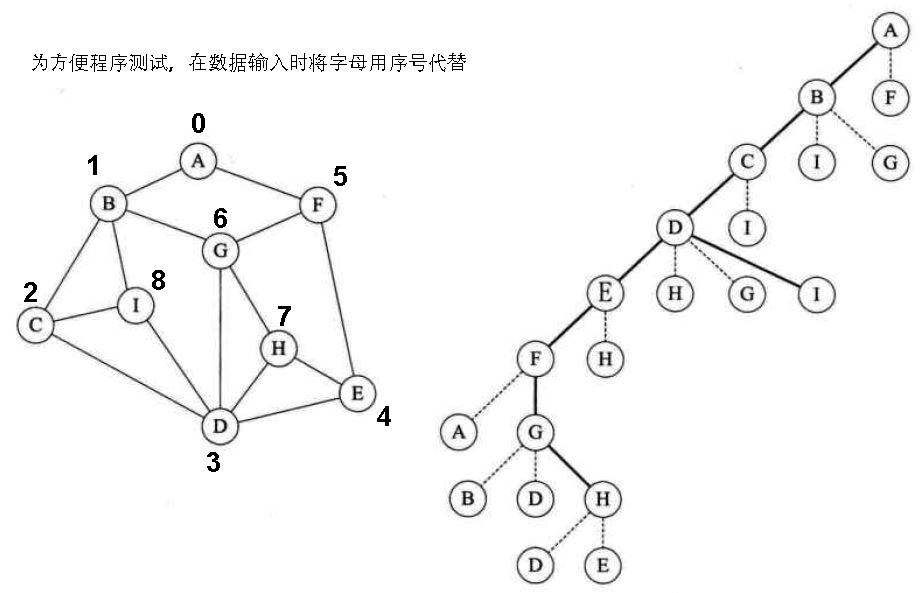## 四、C代码邻接矩阵的DFS实现

/*******************************************************************************************
【DFS】
Author:tmw
date:2018-2-19
********************************************************************************************/
#include <stdio.h>
#include <stdlib.h>
#include <stdbool.h>

#define MAX_VERTEX 100
#define inf 65535  //表示两点之间没有边相连

int visit[MAX_VERTEX];   //标记顶点是否被访问

/**图的邻接矩阵的建立**/
//邻接矩阵数据结构定义
typedef struct Martrix_Graph
{
char vertex[MAX_VERTEX]; //存储顶点信息
int edge[MAX_VERTEX][MAX_VERTEX]; //存储边信息
int vertex_number,edge_number;//存储顶点数和边数
}Martrix_Graph;

void Create_non_direction_martrix_Graph( Martrix_Graph *G )
{
int i,j,k,m;
printf("请输入构造的无向图的顶点数和边数：\n");
scanf("%d %d",&G->vertex_number,&G->edge_number);

printf("请输入无向图顶点信息（如ABCDEF....）：\n");
char ch;
while( ( ch = getchar() != '\n' ) );  //过滤掉前面的\n，防止\n被scanf进去
for(i=0;i<G->vertex_number;i++)
scanf("%c",&G->vertex[i]);

//不相连的顶点之间的权值设为inf，包括顶点自身
//初始化邻接矩阵
for(i=0;i<G->vertex_number;i++)
for(j=0;j<G->vertex_number;j++)
G->edge[i][j] = inf;

//更新无向图边信息
printf("请输入无向图邻接矩阵相连的边信息，相连标记为1\n");
for(k=0;k<G->edge_number;k++)
{
scanf("%d %d %d",&i,&j,&m);
G->edge[i][j] = m;
G->edge[j][i] = G->edge[i][j];//无向图是对称阵
}

//打印邻接矩阵存储信息，检查正确性
printf("---------------------构造出来的无向图邻接矩阵如下---------------------\n");
for(i=0;i<G->vertex_number;i++)
{
for(j=0;j<G->vertex_number;j++)
printf("%d\t",G->edge[i][j]);
printf("\n");
}
}

//从当前第i个顶点出发，DFS遍历
void DFS(Martrix_Graph G, int i)
{
int j;
//标记当前顶点为已访问状态
visit[i] = true;
printf("%c ",G.vertex[i]);

//对与该顶点相连的其他未访问顶点进行DFS
for(j=0;j<G.vertex_number;j++)
{
if( G.edge[i][j]==1 && !visit[j] )
DFS(G,j);
}
}
//对整个图进行DFS
void DFS_Travel(Martrix_Graph G)
{
//初始化visit数组
int i;
for(i=0;i<G.vertex_number;i++)
visit[i] = false;

//整张图的DFS
printf("对该无向图进行DFS的结果如下：\n");
for(i=0;i<G.vertex_number;i++)
{
if( !visit[i] )
DFS(G,i);
}
}

## 五、测试程序及运行结果

int main()
{
printf("测试代码\n");
Martrix_Graph G;
Create_non_direction_martrix_Graph(&G);
DFS_Travel(G);
return 0;
}

运行结果：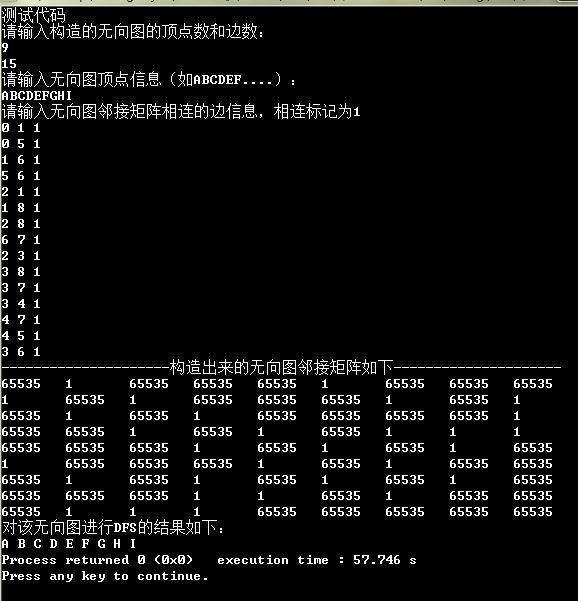展开全文DFS 数据结构
• /*图的存储结构 邻接矩阵用一个一维数组 存放图中所有的顶点信息用一个二维数组 存放边的信息 有向图称为弧 无向图称为边*/#include &lt;iostream&gt;#include &lt;vector&gt;#include &lt;...
/*
图的存储结构  邻接矩阵
用一个一维数组 存放图中所有的顶点信息
用一个二维数组 存放边的信息  有向图称为弧 无向图称为边
*/
#include <iostream>
#include <vector>
#include <string>
#include <deque>

using namespace std;

#define MAXSIZE 32
#define INVAILDVALUE -1
typedef int Node;
//点和边集
struct Graph
{
public:
Graph()
{
nVert = 0;
Vertex.clear();
nEdge = 0;
isDirection = false;
isWeight = false;
edgeInfo = NULL;
}

bool Init(bool bDirection = false);

void print();

void depth();

void depthSearch(int index,bool *visit);

public:
//顶点数
int nVert;
vector<string> Vertex;
//边
int nEdge;
int ** edgeInfo;

//是否有向
bool isDirection;

//是否带权
bool isWeight;

};

bool Graph::Init(bool bDirection)
{
cout << "请输入顶点数" << endl;
cin >> nVert;
getchar();
if (nVert <= 0 || nVert >= MAXSIZE) return false;
string s;
char test;
cout << "请输入顶点信息" << endl;
for (int  i = 0; i < nVert; i++)
{
if (getline(cin, s))
{
Vertex.push_back(s);
}
else
{
sprintf_s(test, "%d", i + 1);
Vertex.push_back(test);
}
}

cout << "请输入边数" << endl;

cin >> nEdge;
//n*(n-1)/2  顶点与边的关系
if (nEdge <= 0 || nEdge >= MAXSIZE || nEdge > nVert*(nVert-1)/2 ) return false;

isDirection = bDirection;

edgeInfo = new int*[nVert];
for (int i = 0; i < nVert; i++)
{
edgeInfo[i] = new int[nVert];
memset(edgeInfo[i], INVAILDVALUE, sizeof(int)*nVert);
}

for (int i = 0; i < nEdge; i++)
{
int Horizontal, Longitudinal,n;
cin >> Horizontal >> Longitudinal>>n;
edgeInfo[Horizontal][Longitudinal] = n;
if (!isDirection)
edgeInfo[Longitudinal][Horizontal] = n;
}
return true;
}

void Graph::print()
{
if (nVert <= 0) return;
for (int i = 0; i < nVert; i++)
{
for (int  j = 0; j < nVert; j++)
{
cout << edgeInfo[i][j] << " ";
}
cout << endl;
}
}

//深度优先遍历
void Graph::depth()
{
cout << "深度优先遍历开始" << endl;
bool visit[MAXSIZE];
memset(visit, true, sizeof(visit));
for (int i = 0; i < nVert; i++)
{
visit[i] = false;
}

for (int i = 0; i < nVert; i++)
{
depthSearch(i, visit);
}
}

void Graph::depthSearch(int index, bool *visit)
{
if (visit[index] )
{
return;
}
cout << "访问" << Vertex[index] << endl;
visit[index] = true;
for (int i = 0; i < nVert; i++)
{
if (!visit[i] && edgeInfo[index][i] != INVAILDVALUE)
{
depthSearch(i, visit);
}
}
}

{
cout << "广度优先遍历开始" << endl;
bool visit[MAXSIZE];
memset(visit, true, sizeof(visit));
memset(visit, false, nVert*sizeof(bool));

for (int i = 0; i < nVert; i++)
{
}
}

deque<int> tDeque;
{
if (visit[index])
{
return;
}
cout << "visit " << Vertex[index] << endl;
visit[index] = true;
for (int i = index + 1; i < nVert; i++)
{
if (!visit[i] && edgeInfo[index][i] != INVAILDVALUE)
{
tDeque.push_back(i);
}
}
while (tDeque.size() > 0)
{
int t = tDeque.front();
tDeque.pop_front();
}
}

int main()
{
while (true)
{
Graph t;
if (t.Init())
{
//t.print();
t.depth();
}
}
return 0;
}
展开全文• 主要介绍了python实现树的深度优先遍历与广度优先遍历,详细分析了树的深度优先遍历与广度优先遍历原理及Python相关实现技巧,需要的朋友可以参考下
• 邻接矩阵，也就是使用二维数组用来存放每个顶点与对应边的关系，例如两个顶点存在边，那么就将这个二维数组对应的下标设置为一个非0值。如下图： 无向图情况： 有向图情况： 邻接矩阵是一种不错的图存储结构，但是...

先附上程序效果：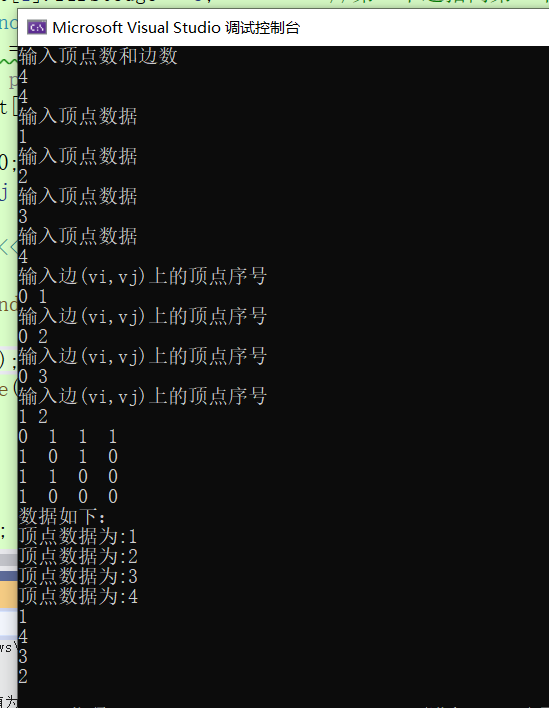## 邻接表：

邻接矩阵，也就是使用二维数组用来存放每个顶点与对应边的关系，例如两个顶点存在边，那么就将这个二维数组对应的下标设置为一个非0值。如下图：
无向图情况：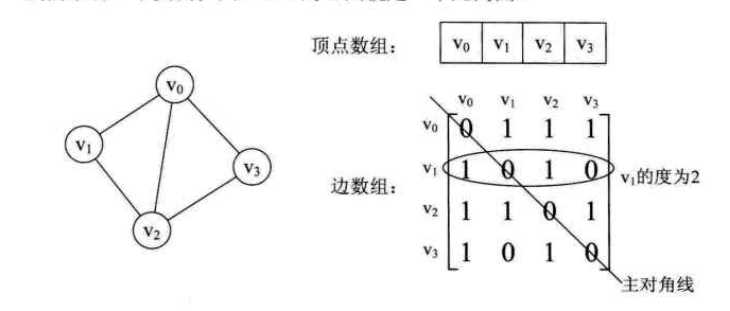有向图情况：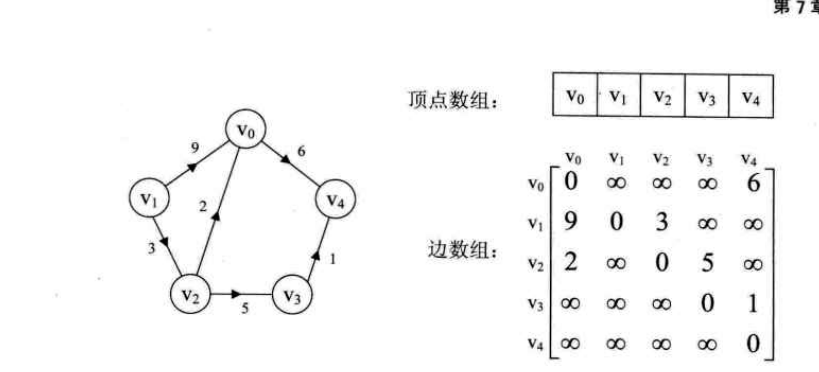邻接矩阵是一种不错的图存储结构，但是对于边数使用较少的图，比较浪费存储空间，
比如下面这种情况：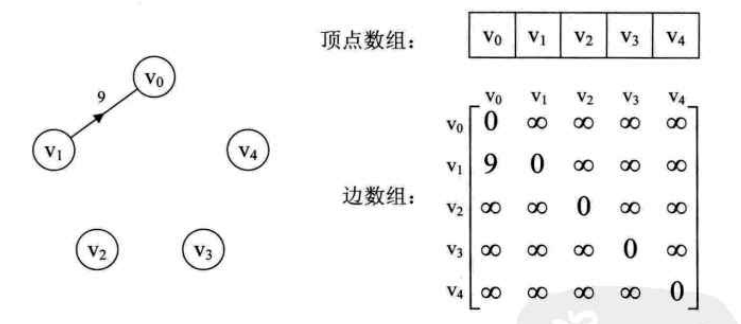而学习线性表的时候我们都知道顺序存储结构浪费空间，所以引出了链式存储结构来节约空间。
因此，同样的我们可以对弧或边使用链式存储结构来避免空间浪费的问题。
于是我们引出了新的图存储结构，邻接表。
我们通过将数组与链表结合来存储一张图，而这种链表与数组相结合的的存储方法称为邻接表。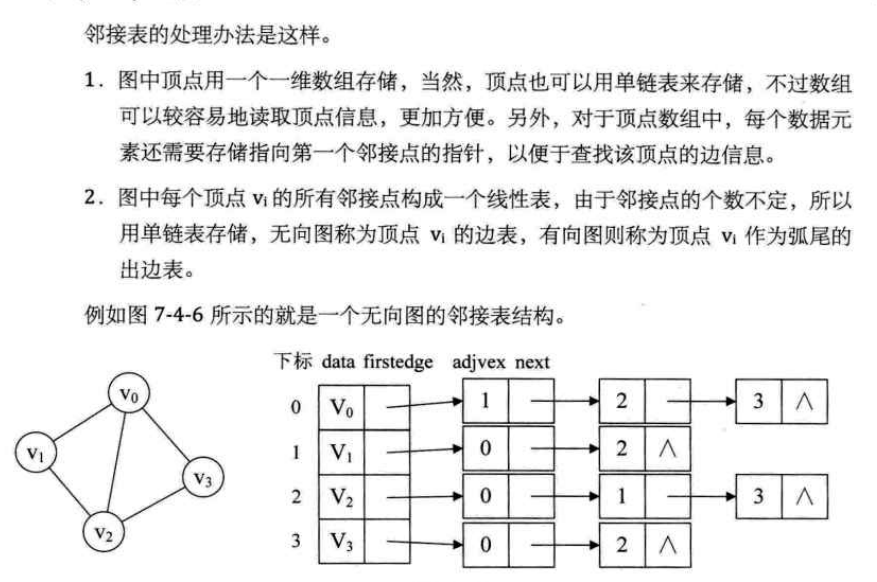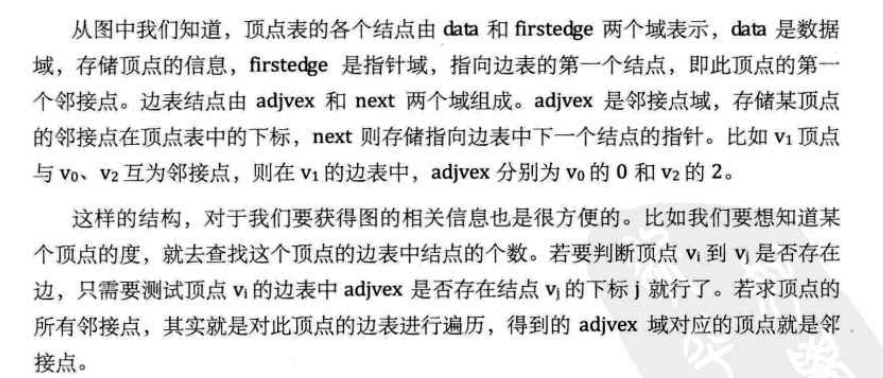那么邻接表大概我们知道是什么样子了，那么实现起来就容易了。
接下来是结构体的定义：

typedef struct Adjvex		//邻接域
{
int weight;		//权值 有没有都可以 看你用的是有向还是无向图
typedef struct Vertxnode	//顶点节点 顶点数组 存放节点以及邻接的顶点的下标指针
{
ElemType data;
typedef struct Graphlist		//邻接表 将顶点数组与顶点数和边数结合
{
int vexnum, edgenum;	//顶点和边数目;
}Graphlist,*p_Graphlist;

int Visited[MAXSIZE] = {0}; //用来表示当前顶点是否被访问 1表示访问 0表示没有访问


对于无向图的构建，我们遵循对称原则，这是由于无向图一条边指向的两个顶点是互相指向对方的，所以在对方的邻接顶点域中都需要将对方的下标存放进来。

void CreateGraphlist(p_Graphlist p)
{
int i, j, k;
Edgenode e;
cout << "输入顶点数和边数" << endl;
cin >> p->vexnum >> p->edgenum;   //输入边数和表数
for (i = 0; i < p->vexnum; i++)		//初始化顶点表
{
cout << "输入顶点数据" << endl;
}
for (i = 0; i < p->vexnum; i++)//二维数组模拟邻接矩阵|0 0 1|
{										    //例如	 |0 0 1|
for (k = 0; k < p->vexnum; k++)			//		 |1 1 0|
{
}
}
for (k = 0; k < p->edgenum; k++)//这个for循环用于输入edgenum个边
{
cout << "输入边(vi,vj)上的顶点序号" << endl; //vi为边尾，vj为边头
cin >> i >> j;			 //输入0，1则代表边尾为v0，边头尾v1
}
for (int i = 0; i < p->vexnum; i++) {  //输出邻接矩阵
for (int j = 0; j < p->vexnum; j++)
{
cout << AdjRectangle[i][j] << "  ";
}
cout << endl;
}
DFSTraverse(p);
}


注意:
这里必须着重讲解一下一个节点有多个邻接点的情况。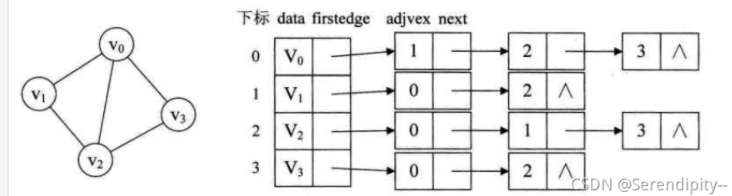图中的每一个节点都不止一个邻接点，那么这些邻接点的next是如何指向下一个邻接点的呢？
光看程序不好想象，那么就让我们debug一下吧。
我们以v0点为例，输入v1与v3两条边(只是为了debug而已，了解一次过程就可以推导下一次的过程)
先建立v0与v1的一条边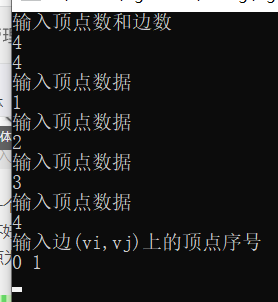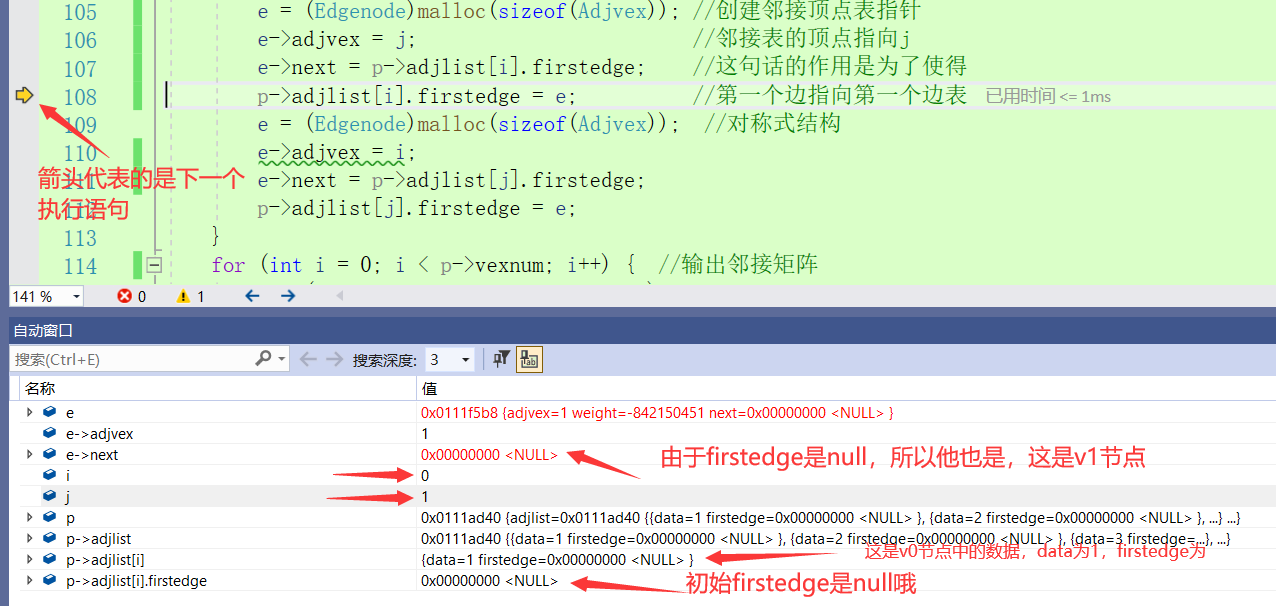中途由于不小心点了一下关闭所以可能和下面图片v1的地址是不一样的，但是要表达的意思一样。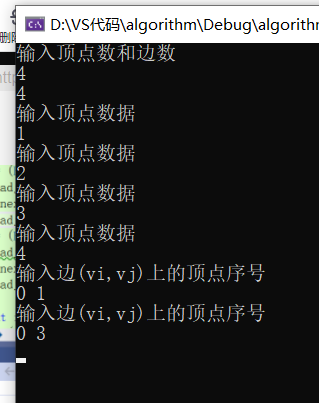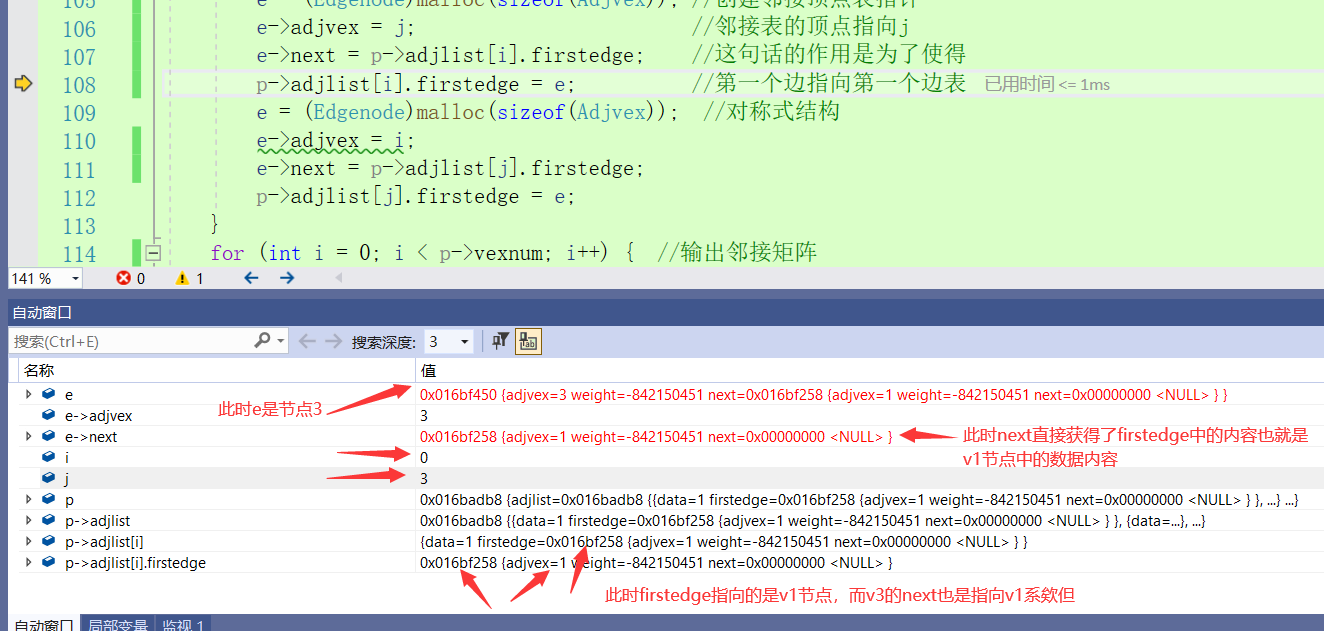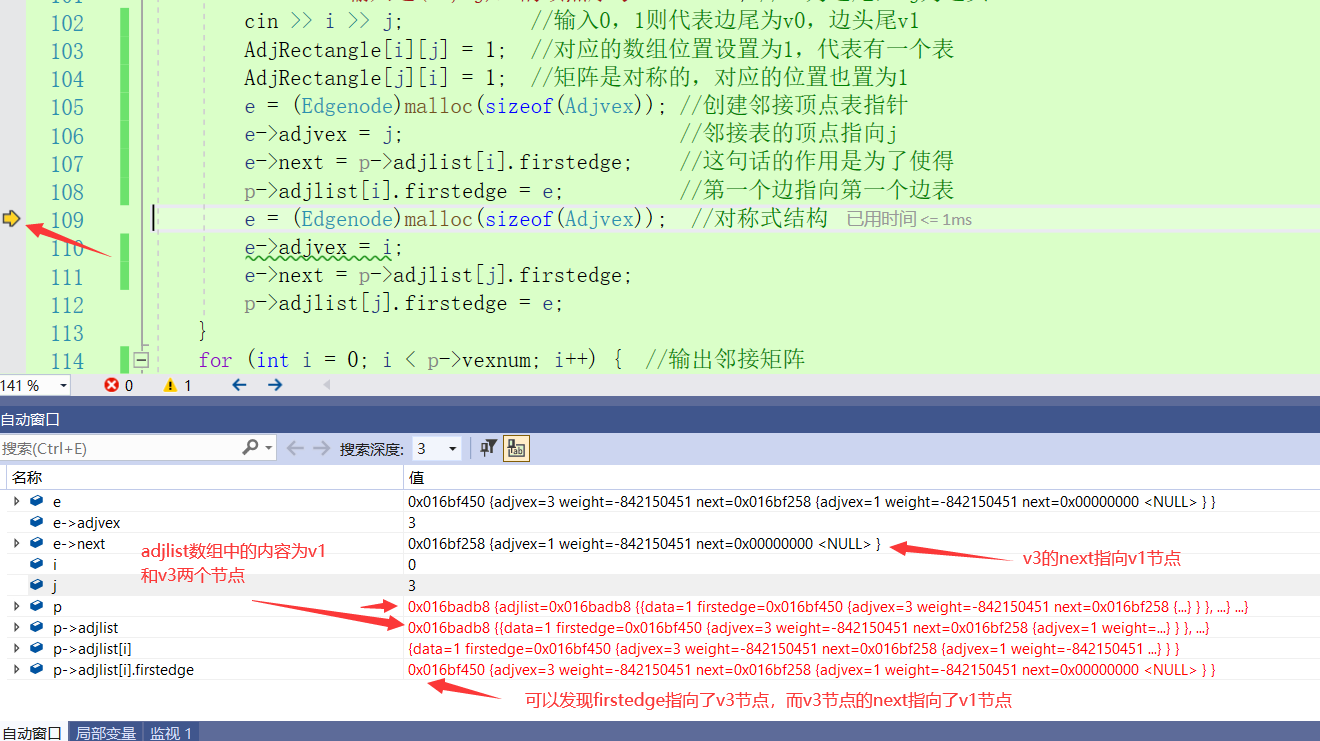可以发现我先输入了v1节点，firstedge会先指向v1节点，而由于firstedge的初始化是NULL，所以v1的next节点是NULL，而之后我输入了v3节点之后，firstedge指向了v3节点，v3节点的next会指向v1节点，这样就形成了一个环路，也就是先输入的反倒在越后面。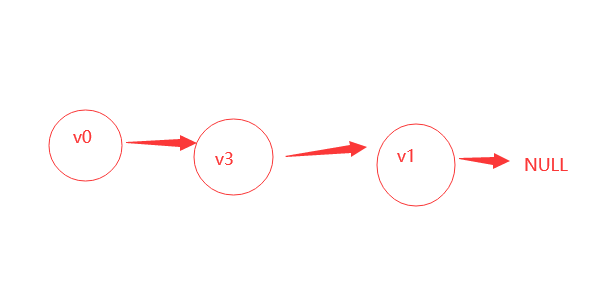那么创建就结束了，比较好理解这个创建，如果实在不理解是怎么创建的，可以进行dubug查看。
那么最后就是重头戏如何遍历整个图了。
我们使用深度优先遍历的方法
那么什么是深度优先遍历？

## 深度优先遍历：

思路大概是从图的某个节点v出发，访问此顶点，然后从v的未被访问的邻接点出发深度优先遍历图，直到所有图中和v有路径的相通的顶点都被访问到。上图中v0节点先访问v1，v1被访问后v1先访问自己的邻接点v2，v2又访问自己的邻接点v3，v3访问自己的邻接点v0，之后发现v0已经被访问过了，那么就从v3退回到v2再从v2退回到v1，v1再退回到v0，这样就形成了深度优先遍历。可以发现这是由递归来实现的。
那么最后直接放代码吧。分为了邻接表和邻接矩阵的递归遍历。

## 代码：

头文件代码：

#pragma once
#include<iostream>
#include <cstdio>
#include<cstdlib>
typedef int Status;
typedef int ElemType;
typedef char cElemType;
#define TRUE 1
#define FALSE 0
#define OK 1
#define ERROR 0
#define MAXSIZE 20
#define make (struct student*)malloc(sizeof(struct student));
using namespace std;



实现代码

#include<algo.h>
{
int weight;		//权值
typedef struct Vertxnode	//顶点节点
{
ElemType data;
typedef struct Graphlist		//邻接表
{
int vexnum, edgenum;	//顶点和边数目;
}Graphlist,*p_Graphlist;

int Visited[MAXSIZE] = {0}; //用来表示当前顶点是否被访问 1表示访问 0表示没有访问
void DFS(p_Graphlist p, int i)//邻接矩阵的深度优先递归遍历
{
int j;
Visited[i] = 1;  //如果访问了该节点则设置为1
cout << "顶点数据为:" << p->adjlist[i].data << endl; //输出当前节点数据
for (j = 0; j < p->vexnum; j++)//循环调用递归
{
if (AdjRectangle[i][j] == 1 && !Visited[j])//如果边表是1且当前节点没有被访问过则调用递归
{
DFS(p, j);//j=0，1，2，3，4...,直到将每一个节点都递归访问了一次之后才结束
}
}
}
void DFSTraverse(p_Graphlist p)
{
int i;
for (i = 0; i < p->vexnum; i++)
{
Visited[i] = 0; //先将所有数据设置为未遍历状态0
}
cout << "数据如下：" << endl;
for (i = 0; i < p->vexnum; i++)//
{
if (!Visited[i])
{
DFS(p,i);
}
}
}
//邻接表的深度优先递归算法
{
Edgenode e;
Visited[i] = 1; //访问了当前节点就设置为1
cout << p->adjlist[i].data << endl; //输出当前数据
while (e)
{
{
}
e = e->next;//让e指向自己的下一个位置
}
}
{
int i;
for (i = 0; i < p->vexnum; i++)
Visited[i] = 0;//初始化访问数组
for (i = 0; i < p->vexnum; i++)
{
if (!Visited[i])
{
}
}
}

void CreateGraphlist(p_Graphlist p)
{
int i, j, k;
Edgenode e;
cout << "输入顶点数和边数" << endl;
cin >> p->vexnum >> p->edgenum;   //输入边数和表数
for (i = 0; i < p->vexnum; i++)		//初始化顶点表
{
cout << "输入顶点数据" << endl;
}
for (i = 0; i < p->vexnum; i++)//二维数组模拟邻接矩阵|0 0 1|
{										    //例如	 |0 0 1|
for (k = 0; k < p->vexnum; k++)			//		 |1 1 0|
{
}
}
for (k = 0; k < p->edgenum; k++)//这个for循环用于输入edgenum个边
{
cout << "输入边(vi,vj)上的顶点序号" << endl; //vi为边尾，vj为边头
cin >> i >> j;			 //输入0，1则代表边尾为v0，边头尾v1
}
for (int i = 0; i < p->vexnum; i++) {  //输出邻接矩阵
for (int j = 0; j < p->vexnum; j++)
{
cout << AdjRectangle[i][j] << "  ";
}
cout << endl;
}
DFSTraverse(p);
}

int main()
{
p_Graphlist p;
p = (p_Graphlist)malloc(sizeof(Graphlist));
CreateGraphlist(p);

}


可以发现邻接矩阵的遍历结果是1 4 3 2，这与我们debug得出的结果是一样的，我们先插入的节点反倒再链表的末尾，后插入的再链表的开头，所以最后插入的v3节点中的数据4最先输出。
那么另一种遍历情况是使用数组判断方法，由于数据是从小到大增加的，所以先插入的节点会先输出，这是邻接矩阵的遍历方法。

## 最后，如果文章对你有帮助的话请点个赞！！！

展开全文c++ 算法 数据结构
• void ShowAdjMat(Mgraph G) //邻接矩阵的显示 { int i,j; printf("The adjacent matrix is:\n"); for(i=0;i;i++) { for(j=0;j;j++) { printf("%d ",G.arcs[i][j].adj); } printf("\n"); } ...数据结构
• 深度优先遍历其实就是一个递归的过程，其详细过程可以表述为：从图中某个顶点v出发，访问此顶点，然后从v的未被访问的邻接点深度优先遍历图，直至图中所有和v有路径相通的顶点都被访问到。（注：此处用文字表述不太...数据结构 dfs
• c语言表述数据结构 无向图用邻接矩阵深度优先遍历程序数据结构
• i++) //初始化邻接矩阵 { for (j = 0; j ; j++) { arc[i][j] = 0; } } for (k = 0; k ; k++) { cout 请输入边的两个顶点的序号;"; cin >> i >> j; arc[i][j] = 1; arc[j][i] = 1; } } template void MGraph::...算法
• 无向图创建邻接矩阵、深度优先遍历和广度优先遍历一、概念解析：（1）无向图：（2）邻接矩阵：二、创建邻接矩阵：三、深度遍历、广度遍历（1）深度遍历概念：（2）广度遍历概念：四、实例展示 一、概念解析： （1）...数据结构 c语言 算法
• 邻接矩阵深度优先遍历和广度优先遍历(无向图) #include<stdio.h> #include<stdlib.h> #include<string.h> #include<queue> #include<iostream> using namespace std; #define ...
• 采用邻接矩阵存储图，进行图的深度优先搜索并输出结果。 1.算法分析： 整个程序分为两部分，第一部分为邻接矩阵存储图，第二部分为图的优先搜索。将两个部分的算法做一个简单的分析即可。 第一部分，邻接矩阵...c语言 递归算法 数据结构
• 由于比较仓促所以直接以代码的形式来讲解，所用为c++中的模板类。· 首先是此代码中用到的头文件：#include&lt;iostream&gt; #include&...· 因为邻接矩阵的广度优先遍历会用到队列，所以我...c++
•数据结构
• 深度优先遍历： 类似与树的前序遍历。从图中的某个顶点v出发，访问此顶点，然后从v的未被访问到的邻接点进行遍历，直到图中所有和v有路径相通的顶点都被访问到（注：优先访问外层节点，访问到无新顶点时，会进行回退...C语言
• Java深度优先遍历和广度优先遍历邻接矩阵算法-数据结构和算法-07-图数据结构 算法
• } } 深度优先遍历阐述 深度优先遍历的思想如下： 因为图的人一顶点都可能与其他顶点相连接，所以图的遍历算法比树的遍历算法要复杂的多。在访问了某个顶点之后，可能沿着某条路径搜索又回到了出发点。因此，为保证...
• #include #include #include /** 邻接矩阵深度优先遍历**/#define MAX 100#define INFINITY 65535// 图结构体typedef struct {char vexs[MAX]; // 顶点的数组，顶点类型为了简单使用charint arc[MAX][MAX]; // 边...
•算法 数据结构
• // 深度优先遍历 void DFS_Traverse(bool adjmatrix[][N], int v0, void (*f)(int)) { bool visited[N] = {true};// v0设为已访问 int stack[N] = {v0};// 栈添加v0 int size = 1; f(v0); while (sizec语言 算法
• 下面简单实现一个邻接矩阵表示的方法的图，以及遍历的两种方式。 Graph.h #pragma once #define MAX_SIZE 30 templateclass T,class E> class Graph { public: Graph(size_t size); virtual ~
• 如果看完本篇博客任有不明白的...常用的图的存储结构主要有以下二种：邻接矩阵邻接表邻接矩阵我们知道，要表示结点，我们可以用一个一维数组来表示，然而对于结点和结点之间的关系，则无法简单地用一维数组来表示了...
• 图的深度优先遍历本质上是一棵树的前序遍历（即先遍历自身，然后遍历其左子树，再遍历右子树），总之图的深度优先遍历是一个递归的过程。 如下图所示，左图是一个图，右图是图的深度优先遍历过程。我们假设从顶点A...java
• //邻接矩阵表示的无向图遍历实现。 #include<iostream> using namespace std; #define MAX_SIZE 100//最大顶点数 。 #define MAX_INT 326564//表示极大值，即 ∞ 。 typedef char Elemtype_A;//定义顶点的数据...数据结构 算法
• 试实现邻接矩阵存储图的深度优先遍历。 函数接口定义： void DFS( MGraph Graph, Vertex V, void (*Visit)(Vertex) ); 其中MGraph是邻接矩阵存储的图，定义如下： typedef struct GNode *PtrToGNode; struct GNode{...c语言 算法
• 邻接矩阵介绍直接说，邻接矩阵是图的一种存储结构。那么图是什么呢？图是一种逻辑结构，和线性结构、树形结构、集合结构一样 是一种逻辑结构用来描述数据对象中的数据元素之间的关系。来看下图的定义：图（Graph）是......